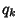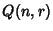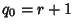## Quota System

A generalization of simple majority voting in which a list of quotasspecifies, according to the number of votes, how many votes an alternative needs to win (Taylor 1995). The quota system declares a tie unless for some, there are exactlytie votes in the profile and one of the alternatives has at leastvotes, in which case the alternative is the choice.

Letbe the number of quota systems forvoters andthe number of quota systems for which, sowhereis the Floor Function. This produces the sequence of Central Binomial Coefficients 1, 2, 3, 6, 10, 20, 35, 70, 126, ... (Sloane's A001405). It may be defined recursively byandwhereis a Catalan Number (Young et al. 1995). The functionsatisfiesfor(Young et al. 1995).satisfies the Quota Rule.

References

Sloane, N. J. A. Sequence A001405/M0769 in An On-Line Version of the Encyclopedia of Integer Sequences.'' http://www.research.att.com/~njas/sequences/eisonline.html and Sloane, N. J. A. and Plouffe, S. The Encyclopedia of Integer Sequences. San Diego: Academic Press, 1995.

Taylor, A. Mathematics and Politics: Strategy, Voting, Power, and Proof. New York: Springer-Verlag, 1995.

Young, S. C.; Taylor, A. D.; and Zwicker, W. S. Counting Quota Systems: A Combinatorial Question from Social Choice Theory.'' Math. Mag. 68, 331-342, 1995.